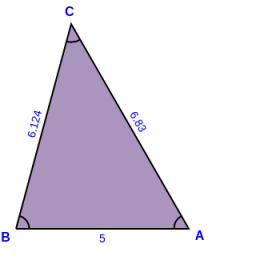# Calculate 72624

The perimeter of the ABC triangle is 19.6 cm. The following applies to the lengths of its sides: a: c = 1: 2, b: c = 5: 6. Calculate the lengths of all sides of the triangle ABC.

a =  3.6296 cm
b =  7.2593 cm
c =  8.7111 cm

### Step-by-step explanation:Did you find an error or inaccuracy? Feel free to write us. Thank you!

Tips for related online calculators
Need help calculating sum, simplifying, or multiplying fractions? Try our fraction calculator.
Check out our ratio calculator.